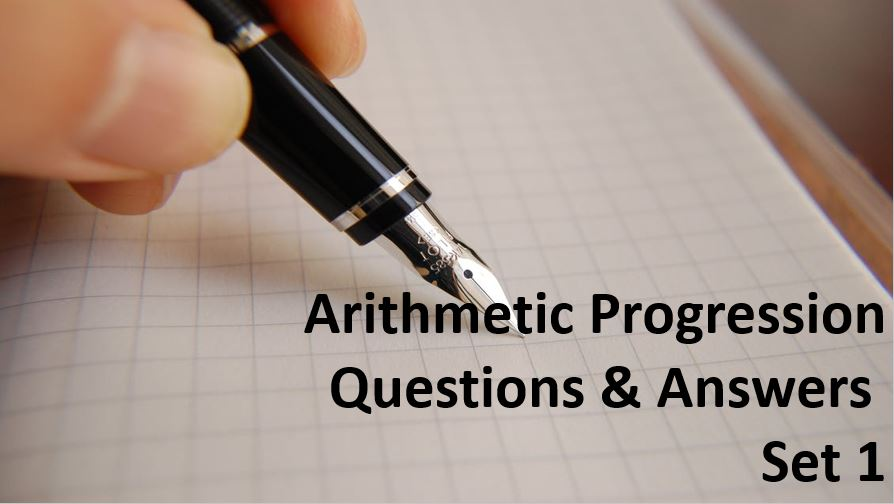Monday, September 27, 2021
Home > Question and Answer > Arithmetic Progression Question And Answer Set 1

# Arithmetic Progression Question And Answer Set 1# Arithmetic Progression Question and Answer Set 1

Hi students, welcome to Amans Maths Blogs (AMB). On this post, you will get the Arithmetic Progression Question and Answer Set 1 is the collection of some important questions. Practice these questions for SSC CGL CHSL CAT NTSEexams etc. It will help you to practice the questions on the topics of maths as linear equations based questions of algebra.

Arithmetic Progression Question and Answer Set 1: Ques No 1

In an AP, 11th term is 5 and 5th term is 11. The 16th term of the AP is

Options:

A. 0

B. 16

C. 11

D. 15

Arithmetic Progression Question and Answer Set 1: Ques No 2

If 13 + 28 + 43 + … to n terms = 68210, then the value of n is

Options:

A. 86

B. 64

C. 95

D. 73

Arithmetic Progression Question and Answer Set 1: Ques No 3

If x, y, z and t are consecutive terms in an arithmetic progression, then the value of (t2 – x2)/(z2 – y2) is ( z2 – y2 is not equal to zero.)

Options:

A. 1

B. 2

C. 3

D. 4

Arithmetic Progression Question and Answer Set 1: Ques No 4

In an AP, 4th term is 7 and 8th term is 15. The value of 10th term of the AP is

Options:

A. 18

B. 19

C. 20

D. 21

Arithmetic Progression Question and Answer Set 1: Ques No 5

In an AP, 4th term is 11 and 7th term is 20. The value of sum of 5th term of the AP is

Options:

A. 20

B. 30

C. 40

D. 50

Arithmetic Progression Question and Answer Set 1: Ques No 6

The sum of the first 20 terms of an AP is identical to the sum of the first 22 terms. If the common difference is −2, find the first term

Options:

A. 23

B. 35

C. 41

D. 62

Arithmetic Progression Question and Answer Set 1: Ques No 7

If the sum of the AP 10, 15, 20, 25, … to n terms is 1045, then the value of nth term is

Options:

A. 100

B. 102

C. 104

D. 106

Arithmetic Progression Question and Answer Set 1: Ques No 8

The number of terms of the arithmetic progression 2, 8, 14, 20, … required to give a sum of 660 is

Options:

A. 13

B. 14

C. 15

D. 16

Get Online Maths Tuition by AMBiPi for Class 8 to 12